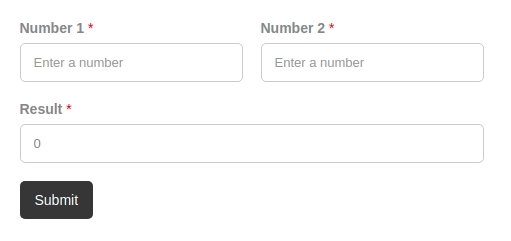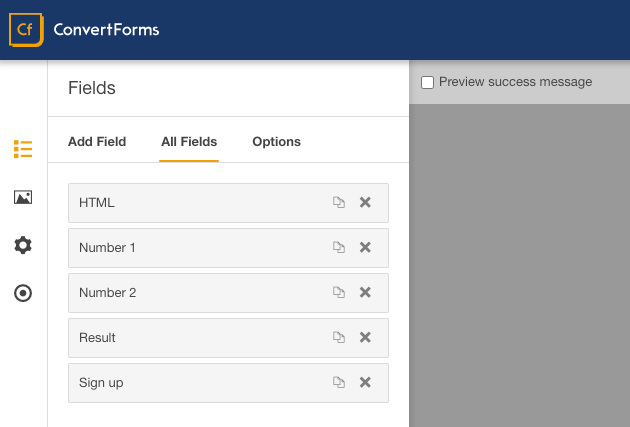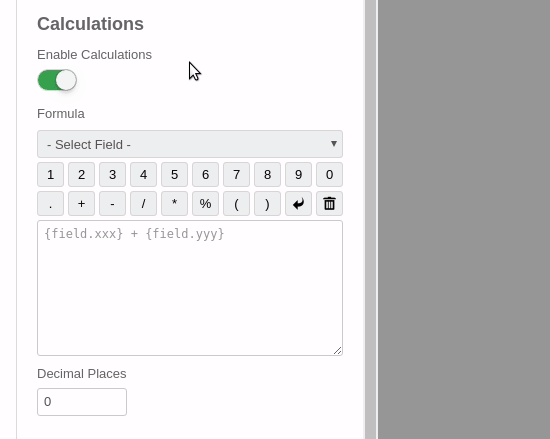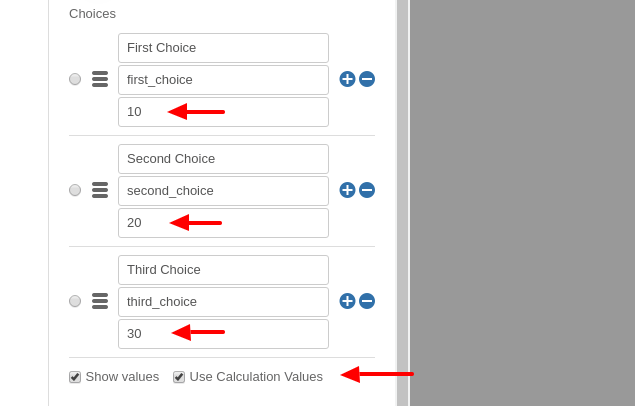# Field Calculations

Published in Convert Forms
Updated 25 Jan, 2023

Wondering how you can calculate a total based on the option selected by the user from your form? With the Convert Forms Calculations Feature you can create forms with dynamically calculated fields to display the calculated values.It includes a Visual Calculation Builder that can help you create both single and complex calculations, for example general calculators, ideal weight calculators, calorie calculators, estimate quotes for hotel booking and rent a car services, price estimation quotes for appointments and services, loan & finance calculators and many more.

## Features

Here's what makes Convert Forms Calculations feature a powerful tool for your forms.

• Easy and visual calculator interface.
• Use hidden fields, text fields, number, drop-down, checkbox or radio buttons.
• Supports math operators like +, -, * or /.
• Use conditional logic in calculations.
• Manage multiple calculation in a form.
• Hide calculated fields.
• Use nested calculations.
• Add a prefix or suffix to calculated value.
• Store calculations in the database.
• Send calculated values via e-mail.
• Create custom calculations with your own formula.

## How to create a Calculation

Let's see how you can easily create a form calculation where you display the sum of 2 fields in a 3rd field as shown in the picture below.### Step 1: Create the form

In order to create a calculation, we need a form with fields of course! In our example, we are going to use just 3 Text or Number fields and a submit button. Let's call the 1st field number1, the 2nd field number2 and the 3rd one for the calculated value, result.### Step 2: Enable Calculations option on the 3rd field

Once the form is ready and the fields are added to the form, click on the 3rd field (where we want to display the calculated value), to open the field settings on the left. Scroll down to find the Calculations section and click to enable it.### Step 3: Create the Calculation Formula

Although our formula is rather simple, we're going to use the Calculation Visual Builder to create it. The steps are shown in the animated movie but also described in the bullets below:• Click on the Field selection dropdown and add the 1st field.
• From the toolbar click to add the '+' math operator to our formula.
• Click on the Field selection dropdown again and add the 2nd field.
• Set the Decimal Places option to 0.
• Optionally, you can set a Prefix and Suffix to your calculation field. Note: The field that will be used must support characters, such as Text Field. If you use Number Field then you wont be able to see the prefix/suffix as it only supports numbers.

Done! Save your form and test it on the front-end.

## Assign Calculcation Values to Dropdowns, Radio Buttons and Checkboxes

Calculation Values are generally used for calculations and they represent the numerical value of an input field. The Calculation Value of a field is different from its Value. A field's Value is what is passed into the final form submission. The Calculation Value is what is used when that field is used in a calculation. This feature is only available in the following fields:

• Dropdown Field
• Checkboxes Field

### How to assign Calculcation Values

To assign calculation values on these fields, select the field and click to open its field settings on the left screen. Scroll down to the Choices section and toggle the Use Calculation Values option to ON.Once the Use Calculation Values option is turnt ON, a 3rd input is shown where you can type in the respective calculation value for each option.

Using the screenshot above as a template, if the user selected both “First Choice” and “Second Choice” on the front end, the saved form data would read “first_choice” and “second_choice” respectively. The Calculation Value for the field would be 30 (10 + 20, the sum of the two choice's calculation values).

## Formula Syntax

### Operator Precedence

The parser accepts a pretty basic grammar. It's similar to normal JavaScript expressions, but is more math-oriented. For example, the ^ operator is exponentiation, not xor.

OperatorDescriptionSyntax
+ Unary plus +y
- Subtract x - y
- Unary minus -y
/ Divide x / y
* Multiply x * y
% Remainder x % y
= Assignment x = y
== Equal x == y
!= Unequal x != y
>= Greater than or equal x >= y
<= Less than or equal x <= y
> Greater than x > y
< Less than x < y
(...) Grouping (x)
! Factorial y!
^ Power x ^ y
and Logical AND x and y
or Logical OR x or y
? : Conditional statements (If-Then-Else) x ? y : z

### Unary operators

The parser has several built-in "functions" that are actually unary operators. The primary difference between these and functions are that they can only accept exactly one argument, and parentheses are optional. With parentheses, they have the same precedence as function calls, but without parentheses, they keep their normal precedence (just below ^). For example, sin(x)^2 is equivalent to (sin x)^2, and sin x^2 is equivalent to sin(x^2).

OperatorDescription
abs x Absolute value (magnatude) of x.
acos x Arc cosine of x.
acosh x Hyperbolic arc cosine of x.
asin x Arc sine of x.
asinh x Hyperbolic arc sine of x.
atan x Arc tangent of x.
atanh x Hyperbolic arc tangent of x.
ceil x Ceiling of x — the smallest integer that’s >= x.
cos x Cosine of x.
cosh x Hyperbolic cosine of x.
exp x e^x (exponential/antilogarithm function with base e).
floor x Floor of x — the largest integer that’s <= x.
length x String length of x.
ln x Natural logarithm of x.
log x Natural logarithm of x (synonym for ln, not base-10).
log10 x Base-10 logarithm of x.
not x Logical NOT operator.
round x X, rounded to the nearest integer, using "gradeschool rounding".
sin x Sine of x.
sinh x Hyperbolic sine of x.
sqrt x Square root of x. Result is NaN (Not a Number) if x is negative.
tan x Tangent of x.
tanh x Hyperbolic tangent of x.
trunc x Integral part of a X, looks like floor(x) unless for negative number.

### Pre-defined functions

Besides the "operator" functions, there are several pre-defined functions.

FunctionDescription
random(n) Get a random number in the range [0, n). If n is zero, or not provided, it defaults to 1.
min(a,b,…) Get the smallest (minimum) number in the list.
max(a,b,…) Get the largest (maximum) number in the list.
hypot(a,b) Hypotenuse, i.e. the square root of the sum of squares of its arguments.
pyt(a, b) Alias for hypot.
pow(x, y) Equivalent to x^y. For consistency with JavaScript's Math object.
atan2(y, x) Arc tangent of x/y. i.e. the angle between (0, 0) and (x, y) in radians.
if(c, a, b) Function form of c ? a : b
roundTo(x, n) Rounds x to n places after the decimal point.

### Constants

There's also a number of pre-defined constants that can be used in calculations. These are shown in the table below:

ConstantDescription
E The value of E represents the base of natural logarithms, e, approximately 2.718.
PI The value of PI represents the ratio of the circumference of a circle to its diameter, approximately 3.14.
true Logical true value
false Logical false value

## How to display a Calculation in a HTML Field

Besides that you can display the result of a calculation in an input field, you can also display the evaluated result of a calculation in any HTML element like a <span>, <p>or a <div> tag. Let's see how this is possible.

Add an HTML field to your form from the Fields section. Once added, open the HTML field settings and see the Default Value option with the rich text editor. There, click the source code button found in the editor's toolbar to open the Source Code dialog. Here, we will need to add an HTML element with specific HTML data attributes that will act as a placeholder for the calculation result.

Using the example described in the How to create a Calculation section, let's say we want to display the sum of the 2 fields in a <span> element also. To make that happen, we will need to add the following HTML code:

``Sum: <span data-calc="{field.number1} + {field.number2}">0</span>``

In the code above, we define the same formula we've used in the Hidden field using the data-calc attribute while we are also setting the default text to 0. When a value of any of our form fields change, the calculation we will run again and the result will be displayed within our HTML element.

It's recommended to define a default text in the elements you're using for calculations. Doing otherwise, the editor is very likely to strip the HTML element if it has no text.

### Using the Calculated Value from another field

Although our calculation works, it's not that efficient. The sum of the 2 fields is already calculated in the Hidden field since it's ordered before the HTML field. Wouldn't be better to pull the already calculated value from the Hidden field and display it in our HTML element instead of running the same calculation over and over again? For the sake of simplicity, our code can be rewritten as:

``Sum: <span data-calc="{field.result}">0</span>``

In the updated code, we set the calculation formula to match the value of the result field which at the time the calculations are triggered it will already have the calculated value since the calculations are executed from top to bottom. The behavior of using the calculated value of another field in a calculation is known as Calculation Nesting.

### Supported HTML Attributes

Below you can find a list of the HTML attributes you can use to customize an inline calculation.

Property NameDescriptionType
data-calc The formula syntax Text
data-precision Define the decimal places precision Number
data-prefix Add text at the beginning of the calculated value Text
data-suffix Add text at the end of the calculated value Text
data-thousand_separator Make big numbers more readable by separating thousands with a comma. Text
data-decimal_separator Make big numbers more readable by separating decimals with a dot. Text

### How to use data from the database in a calculation?

To include database information in a calculation, you'll need to add a Hidden field to the form and pre-populate its value using PHP during the Form Prepare event. To learn how you can make that happen see the PHP Scripts guide. Then, you will be able to use your hidden field in the calculation.

### How to use a query string parameter in a calculation?

To use a query string parameter in a calculation, you need to first add a Hidden field to the form, set its value with the Query String Smart Tag {querystring.PARAM} and then use that hidden field in the calculation.

### When are the calculations processed?

Calculations are processed in the browser during page load and every time a field value changes. This way we ensure the results are updated live providing a great experience to the user. For security reasons, the calculations are re-run during submission to prevent tampering and illegal field manipulation.

### In which order are evaluated the formulas associated to calculated fields?

Each time a field value changes an event is fired to re-calculate all the fields from top to bottom so every value is added consecutively.

• Product A: 15 | Price = 15
• Product B: 20 | Price = 35
• Product C: 10 | Price = 45
• Price = 45

### How to use conditional statements in a calculation?

Let's say you have created a form to sell a book and you'd like to give a special 30% discount when the quantify is 3 or more. There are 2 ways to do so:

1. Using the ternary operator (x ? y : z)

``{field.qty} >= 3 ? 30 : 0``

2. Using the if built-in method if(condition, value if true, value if false) which accepts 3 arguments.

``if({field.qty} >= 3, 30, 0)``

### Can I perform calculations with dates?

Unfortunately, this is not supported yet. It's something on our to-do list but there's no ETA on when this feature will be available. Until then, you can use a workaround with a small JavaScript snippet that can help you calculate the difference between 2 dates fields.

#### Javascript workaround for calculating the difference between 2 dates

• Add 2 Date / Time fields to your form and add the classes fp_check_in and fp_check_out respectively to the CSS Class setting.
• Add a Hidden field to your form and set the field key to number_of_days to store the days between the 2 dates.
• Place the following Javascript code into your form's Custom Code option which can be found under the Form -> Design -> Advanced panel.
``````setTimeout(function(){
var fp_check_in = flatpickr(".fp_check_in input.flatpickr-input", {
disableMobile: true,
onClose: function(checkin_date, dateStr, instance){
var checkout_date = document.querySelector(".fp_check_out input.flatpickr-input")._flatpickr;
checkout_date = checkout_date.selectedDates;
if (checkin_date != '' && checkout_date != '')
{
calculateDays(checkin_date, checkout_date);
}
}
});

var fp_check_out = flatpickr(".fp_check_out input.flatpickr-input", {
disableMobile: true,
onClose: function(checkout_date, dateStr, instance){
var checkin_date = document.querySelector(".fp_check_in input.flatpickr-input")._flatpickr;
checkin_date = checkin_date.selectedDates;
if (checkin_date != '' && checkout_date != '')
{
calculateDays(checkin_date, checkout_date);
}
}
});

function calculateDays(check_in, check_out) {
var date1 = new Date(check_in);
var date2 = new Date(check_out);

// To calculate the time difference of two dates
var Difference_In_Time = date2.getTime() - date1.getTime();

// To calculate the no. of days between two dates
var Difference_In_Days = Math.ceil(Difference_In_Time / (1000 * 3600 * 24));

if (Difference_In_Days < 0) {
Difference_In_Days = 0;
}

document.querySelector('input[name="cf[number_of_days]"]').value = Difference_In_Days;
}
}, 500);``````
Note: Don't forget to wrap the Javascript code with the <script> tag in order to be parsed by the browser.

After finishing with the above guide, you can use the hidden field num_of_days in your Field Calculations.

### Why does the field contain the previous value than the currently calculated one?

If you are using JavaScript to populate a hidden field which you then use in another field's calculation, you may find that the latter does not update its value as displays the previous value of the hidden field. This is caused due the calculations not running after you update the hidden field. You will need to trigger the calculations right after you change the value of the hidden field.

Below you can find how to trigger the calculations to re-run when you update a field:

``````var el = document.querySelector("#input");
el.value = 70;
el.dispatchEvent(new Event('input', { 'bubbles': true }));``````

You can also trigger the calculations on the form itself:

``````var el = document.querySelector("#input");
el.value = 70;
el.closest("form").dispatchEvent(new Event('input'));``````